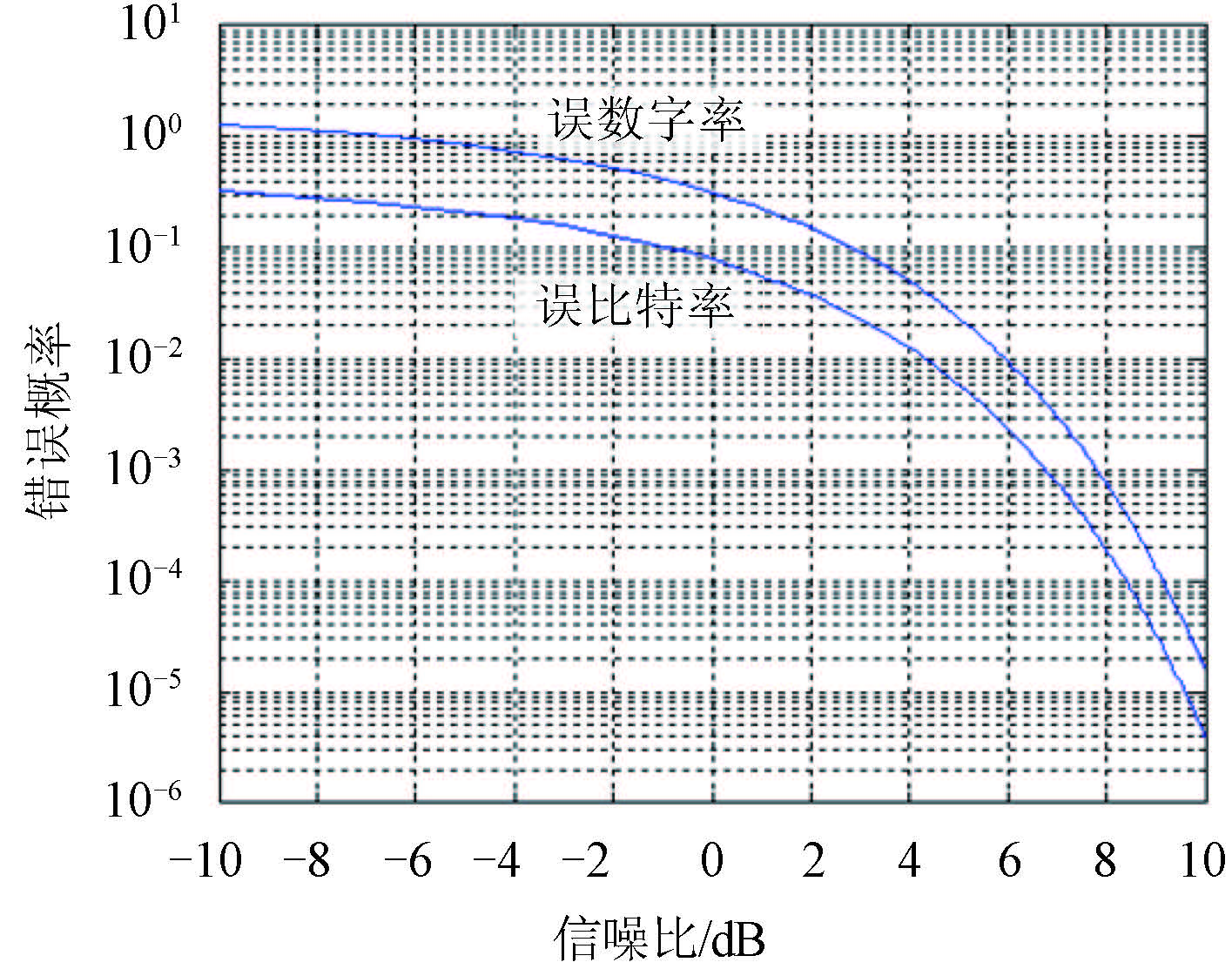﻿ 甚低频通信的MSK与MFSK性能比较分析
 舰船科学技术2016, Vol. 38Issue (6): 151-153PDF

1. 中国人民解放军 91469 部队, 北京 100841 ;
2. 中国人民解放军 91960 部队, 广东 汕头 515000

The performance comparison between MSK and MFSK modulation of VLF communication
REN Xi-chuang1, YAN Shuo2
1. No. 91469 Unit of PLA, Beijing 100841, China ;
2. No. 91960 Unit of PLA, Shantou 515000, China
Abstract: This project carry on comparative analysis about VLF communications method of 16FSK and MSK from aspects of beer and spectrum effectiveness, giving the result about using the communications modulation of 16FSK may utilize the advantages of its own and reduce communications rate to exchange communications distance, depth and quality. This project provides scientific and reasonable theoretical support on submarine communication's depth and long distance modification selection of assessment. Thirdly, this project provides the mode of connection and the function about communication terminal of 16FSK and existing submarine communication equipment at the first time, having a strong guiding significance for engineering implementation.
Key words: VLF     MSK     MFSK
0 引 言

1 甚低频 MSK 与 MFSK 性能仿真

 ${P_e} = \frac{1}{{\sqrt {2\pi } }}\int\limits_{\sqrt {\frac{{2{E_b}}}{{{n_0}}}} }^\infty {{e^{ - {{{x^2}} \mathord{\left/ {\vphantom {{{x^2}} 2}} \right. } 2}}}{\text{d}}x} = Erfc\left( {\sqrt {\frac{{2{E_b}}}{{{n_0}}}} } \right)\text{。}$ (1)

 ${P_z} = 1 - {\left( {1 - {P_e}} \right)^4} \approx 4{P_e}\text{。}$ (2)

 $\begin{array}{l} {P_e} = \displaystyle\frac{1}{{\sqrt {2\pi } }}\left( {\int\limits_0^\infty {{e^{ - {{{x^2}} \mathord{\left/ {\vphantom {{{x^2}} 2}} \right. } 2}}}{\text{d}}x} - \int\limits_0^{\sqrt {{{2{E_b}} \mathord{\left/ {\vphantom {{2{E_b}} {{n_0}}}} \right. } {{n_0}}}} } {{e^{ - {{{x^2}} \mathord{\left/ {\vphantom {{{x^2}} 2}} \right. } 2}}}{\text{d}}x} } \right) = \\[15pt] 0.5 - \displaystyle\frac{1}{{\sqrt {2\pi } }}\int\limits_0^{\sqrt {{{2{E_b}} \mathord{\left/ {\vphantom {{2{E_b}} {{n_0}}}} \right. } {{n_0}}}} } {{e^{ - {{{x^2}} \mathord{\left/ {\vphantom {{{x^2}} 2}} \right. } 2}}}{\text{d}}x}\text{。} \end{array}$ (3)图 1 MSK 误码率与信噪比的关系曲线 Fig. 1 The relationship between MSK error rate and SNR

2 甚低频 MSK 与 MFSK 应用分析

 ${P_{s,MSK}} = {Q_{MSK}}{n_0}/{T_{b,MSK}} \text{。}$ (4)

 $\frac{{{P_{s,16FSK}}}}{{{P_{S,msk}}}} = \frac{{{Q_{16FSK}}}}{{{Q_{MSK}}}}\frac{{{T_{b,MSK}}}}{{{T_{b,16FSK}}}} \text{。}$ (5)表 1 信息速率不同条件下所需发信功率的比值 Tab.1 The ratio of transmitting power under different information rate

3 结 语

  潘威炎. 长波超长波极长波传播[M]. 成都: 电子科技大学出版社, 2004 .  TRANTER W H, SHANMUGAN K S, RAPPAPORT T S, et al. Principles of communication systems simulation with wireless applications[M]. New York: Pearson Education, 2004 .  孟庆辉, 王永斌, 赵志礼. 潜艇周围影响超低频通信的电磁噪声来源及特征分析[J]. 舰船科学技术 , 2009, 31 (11) :66–69. MENG Qing-hui, WANG Yong-bin, ZHAO Zhi-li. The source and characteristics of extremely low frequency noise affecting communications around the submarine[J]. Ship Science and Technology , 2009, 31 (11) :66–69.  李光明, 宋裕农, 蔡广友. 甚低频通信信道建模与仿真[J]. 通信技术 , 2010, 43 (6) :41–42. LI Guang-ming, SONG Yu-nong, CAI Guang-you. Modeling and simulation of VLF channel[J]. Communications Technology , 2010, 43 (6) :41–42.  张俊昌, 曹海鹏, 谢家祥. 甚低频对潜通信数据压缩编码技术研究[J]. 通信技术 , 2008, 41 (12) :262–264. ZHANG Jun-chang, CAO Hai-peng, XIE Jia-xiang. Research of data compress and encoding technique in VLF communication for submarine[J]. Communications Technology , 2008, 41 (12) :262–264.  张文娟, 王永斌, 付天晖. 甚低频/低频通信中大气噪声仿真研究[J]. 舰船电子工程 , 2006, 26 (6) :123–126. ZHANG Wen-juan, WANG Yong-bin, FU Tian-hui. Simulation study on atmospheric noise in VLF/LF communication[J]. Ship Electronic Engineering , 2006, 26 (6) :123–126.  付贞, 温东, 姜波, 等. 甚低频大气噪声中的MSK通信系统仿真[J]. 舰船电子工程 , 2010, 30 (1) :98–101. FU Zhen, WEN Dong, JIANG Bo, et al. Simulation of MSK communication system in VLF atmospheric noise[J]. Ship Electronic Engineering , 2010, 30 (1) :98–101.  任席闯, 左丽芬, 李树明, 等. 基于GIS的超低频通信服务区的预测[J]. 舰船科学技术 , 2013, 35 (4) :117–119. REN Xi-chuang, ZUO Li-fen, LI Shu-ming, et al. The prediction of SLF communication coverage based on GIS[J]. Ship Science and Technology , 2013, 35 (4) :117–119.  MALLIK R K, GOGOI A K. An optimum detector for coherent M-ary signaling in the presence of impulsive noise[C]//Proceedings of the 2000 IEEE international conference on personal wireless communications. Hyderabad:IEEE, 2000:363-367.